# 实现模型周边感应功能

## 需求目标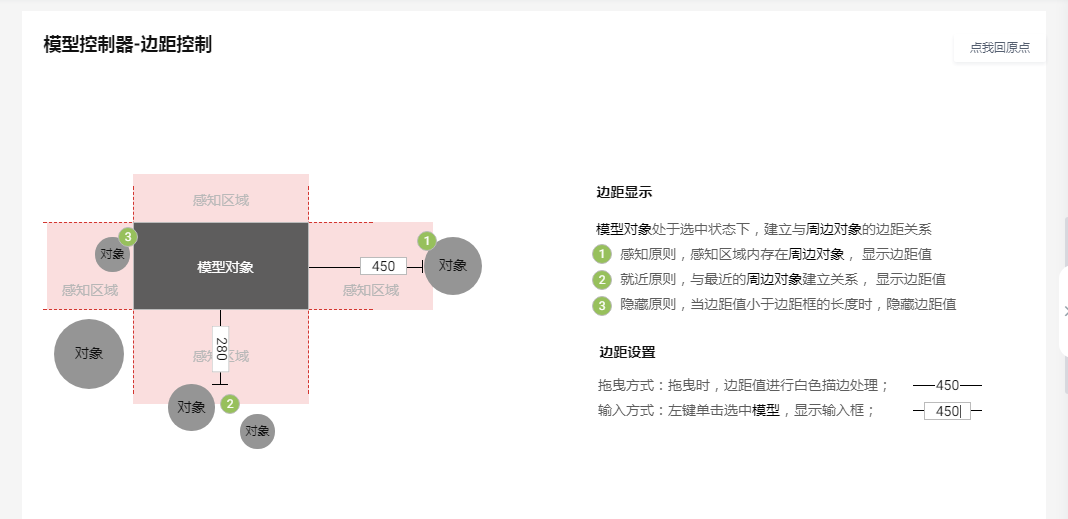## 方案思路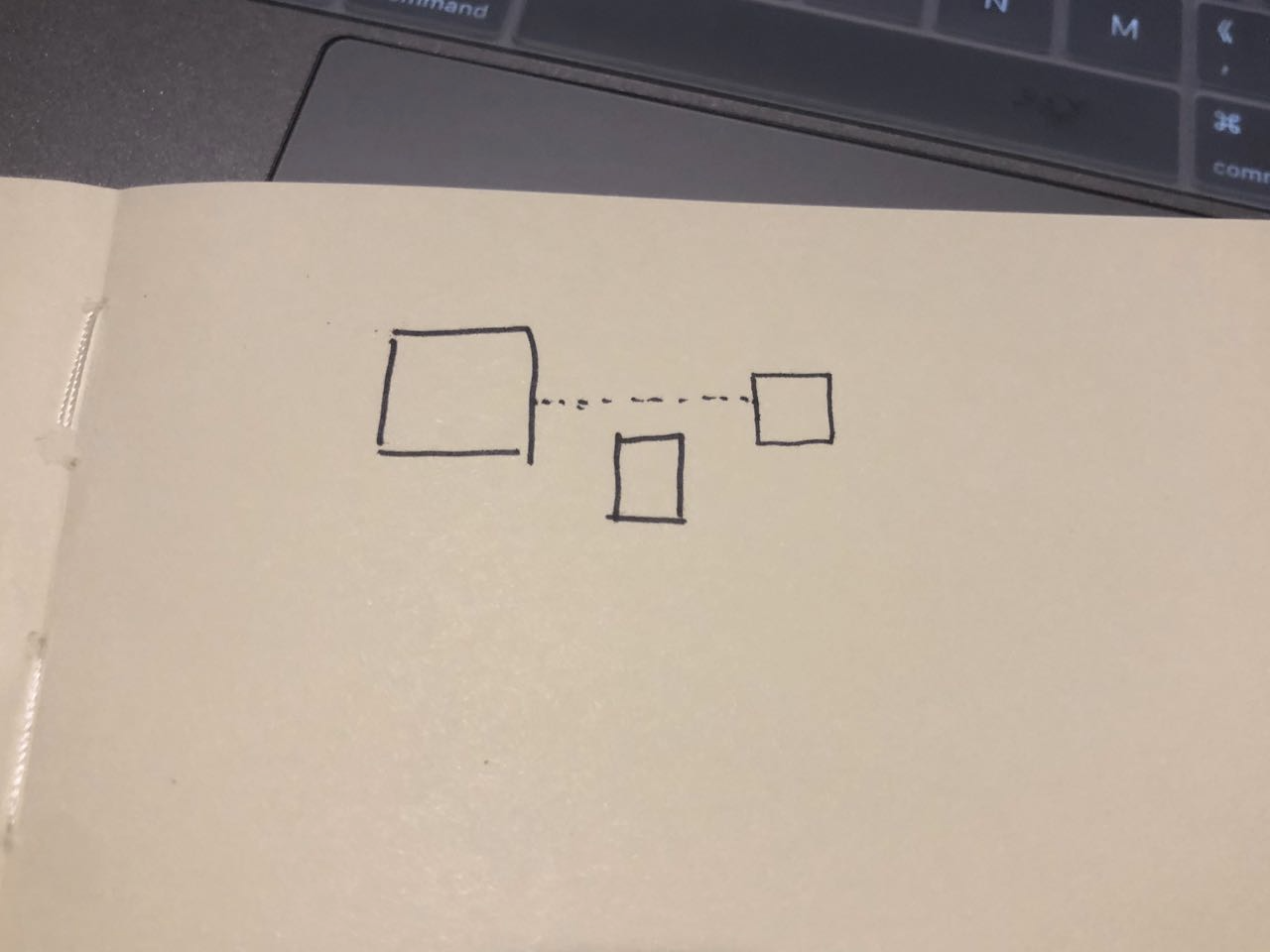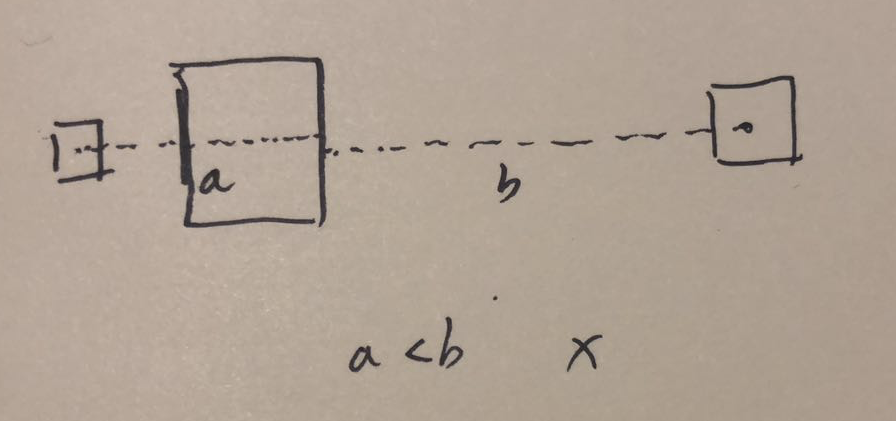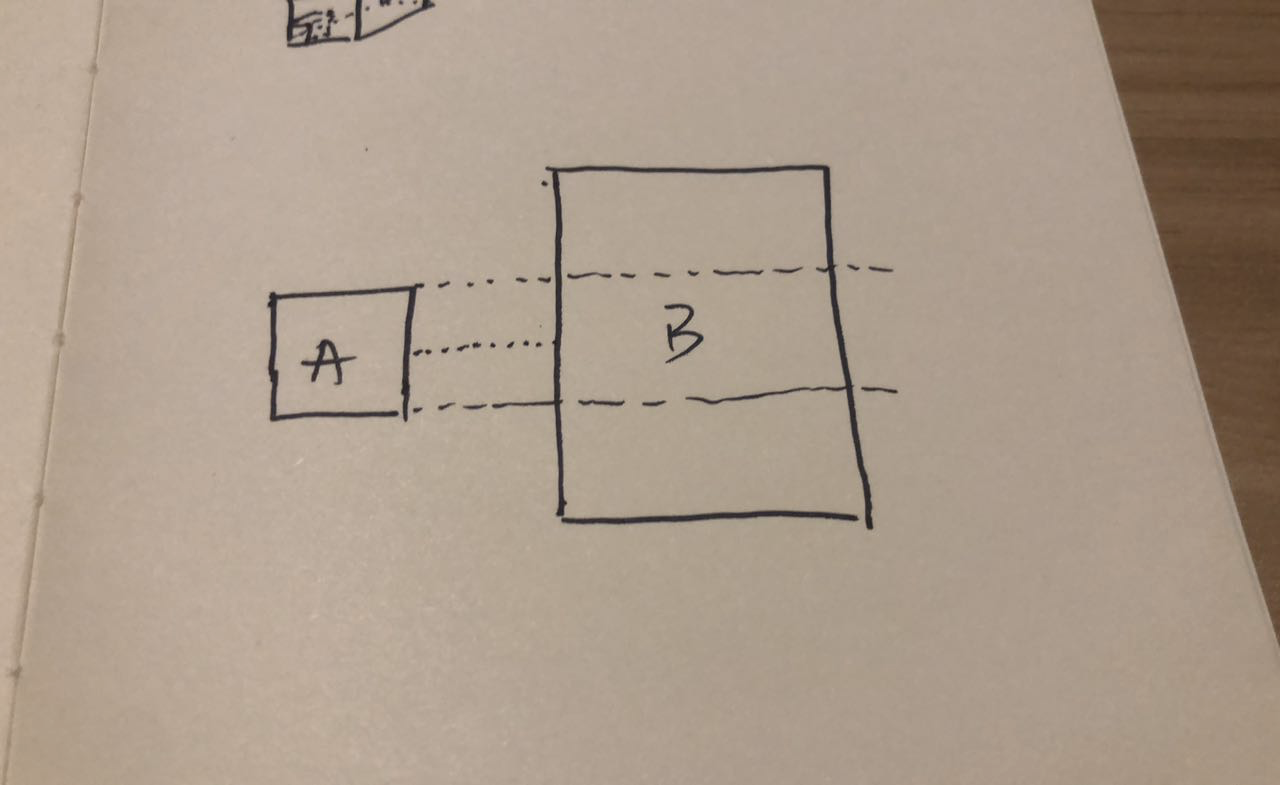- 找到当前选中模型的boundingBox2D的四条边
- 遍历四条边，查找每一条边的最近模型包含
- 找到所有模型（可过滤为当前房间的所有模型）
- 遍历所有模型boundingBox2D
- 遍历boundingBox2D的四条线段
- 是与比较线段平行？
- 是在比较线段的感应范围内？
- 横向线段比较x范围
- 纵向线段比较y范围
- .........


 显然这样的算法是普遍的，这里不做具体展开，所以在封装了Polygon2D.insterectPolygon(polygon:Polygon2D):Polygon2D

- 找到选中模型的boundingBox2D的四个射线感应多边形（扩大多边形范围）(图1)
- 获取所有需要查找的范围集合，包含模型和墙体(后面统一称为compares)(图2)
- 遍历四个方向的感应多边形
- 遍历查找范围集合，从compares中查找到最近的一点
- 过滤未相交的compare(图3)
- 将所有过滤后的polygon集合展开为所有多边形点的集合=>points
- 找出points与选中模型最近的点(图4)
- 找到的最近一点与当前方向一边的垂足
- 连接垂足与最近点成线段(图5)
- 平移线段到正确点的位置(图6)
- 绘制找到的四条线段
- 后续对线段长度标注、根据线段方向与设置大小，改变模型位置...


### 过程图：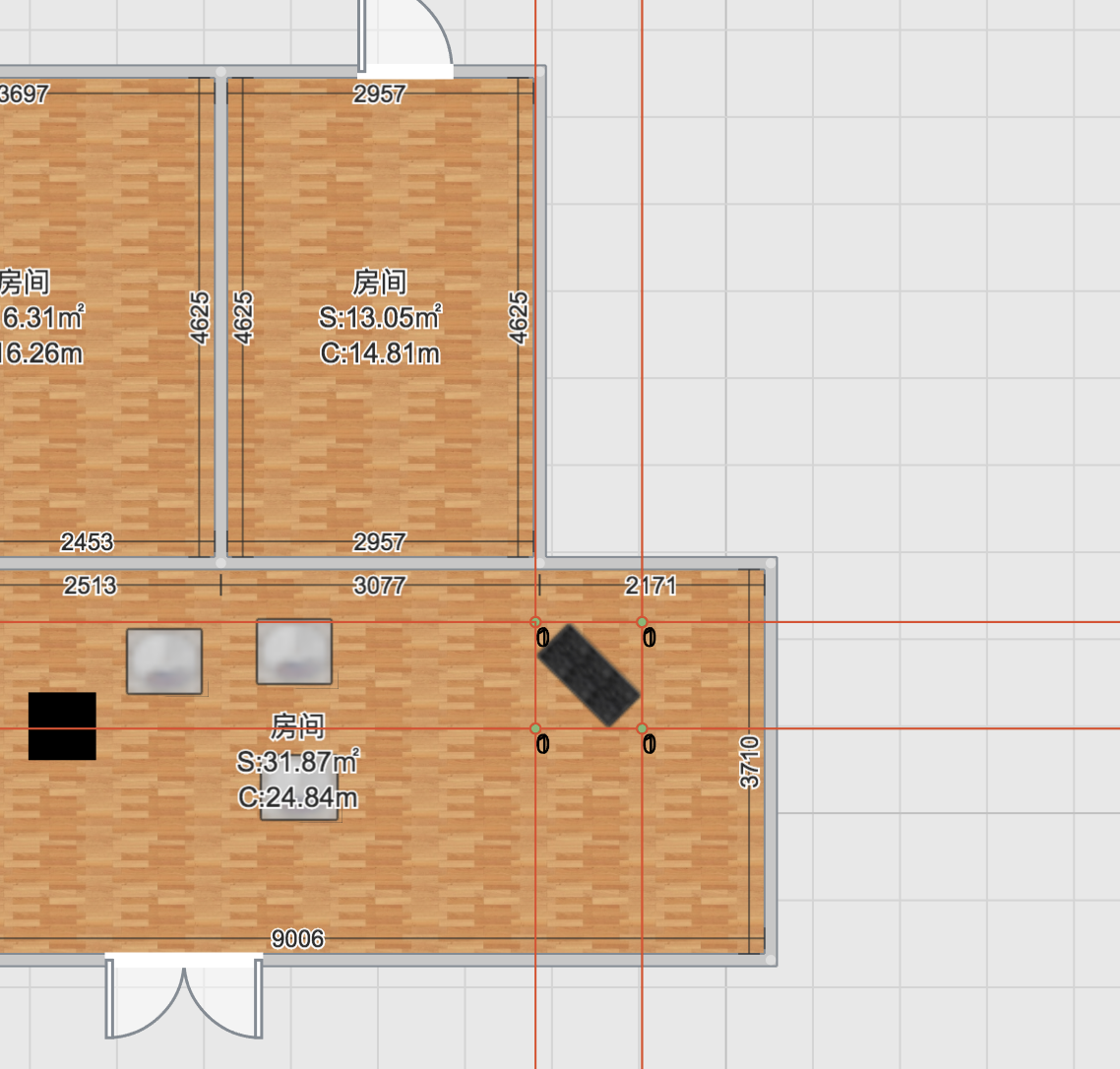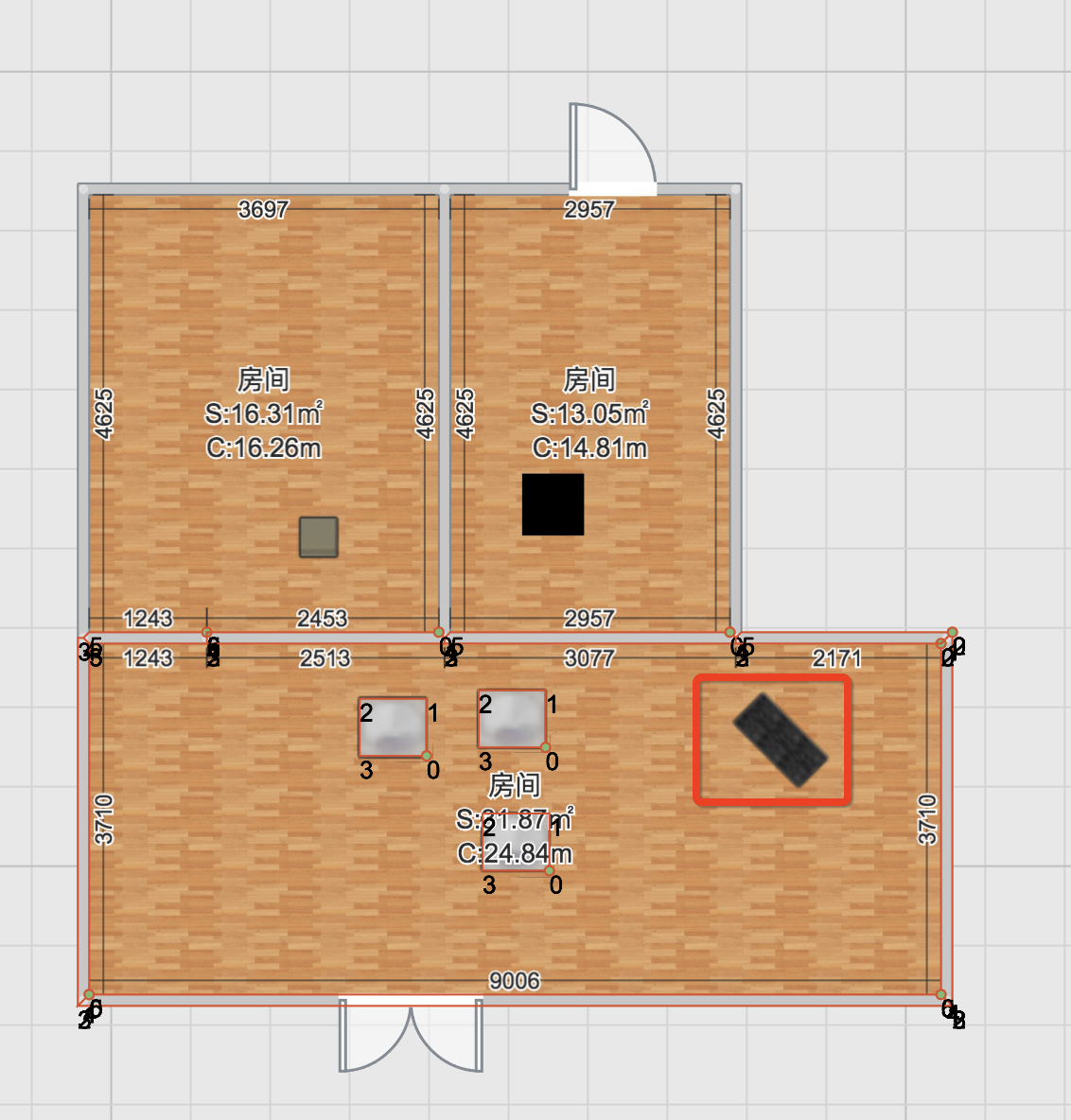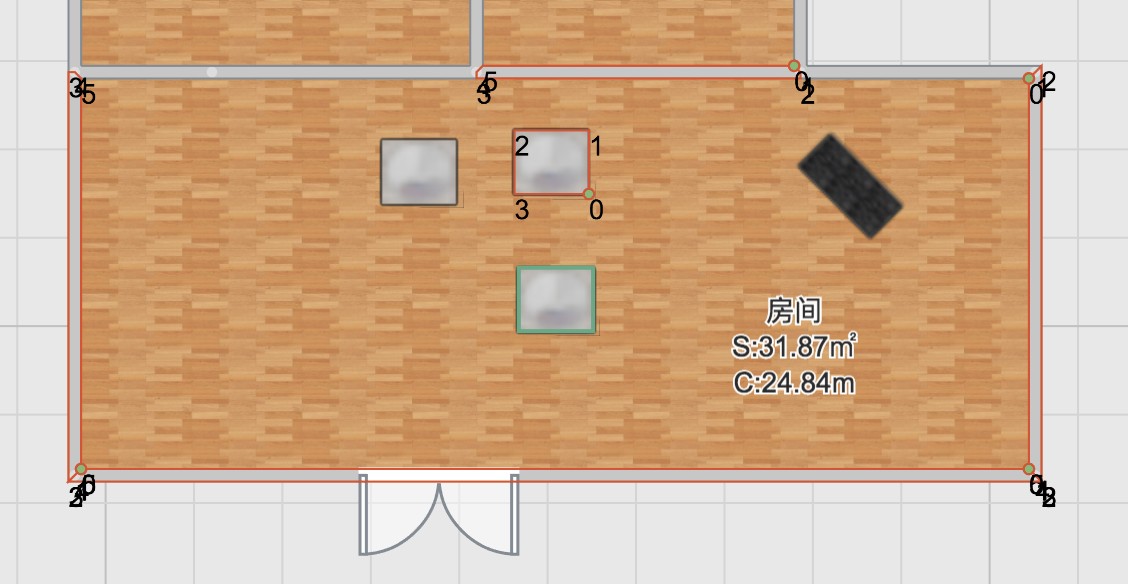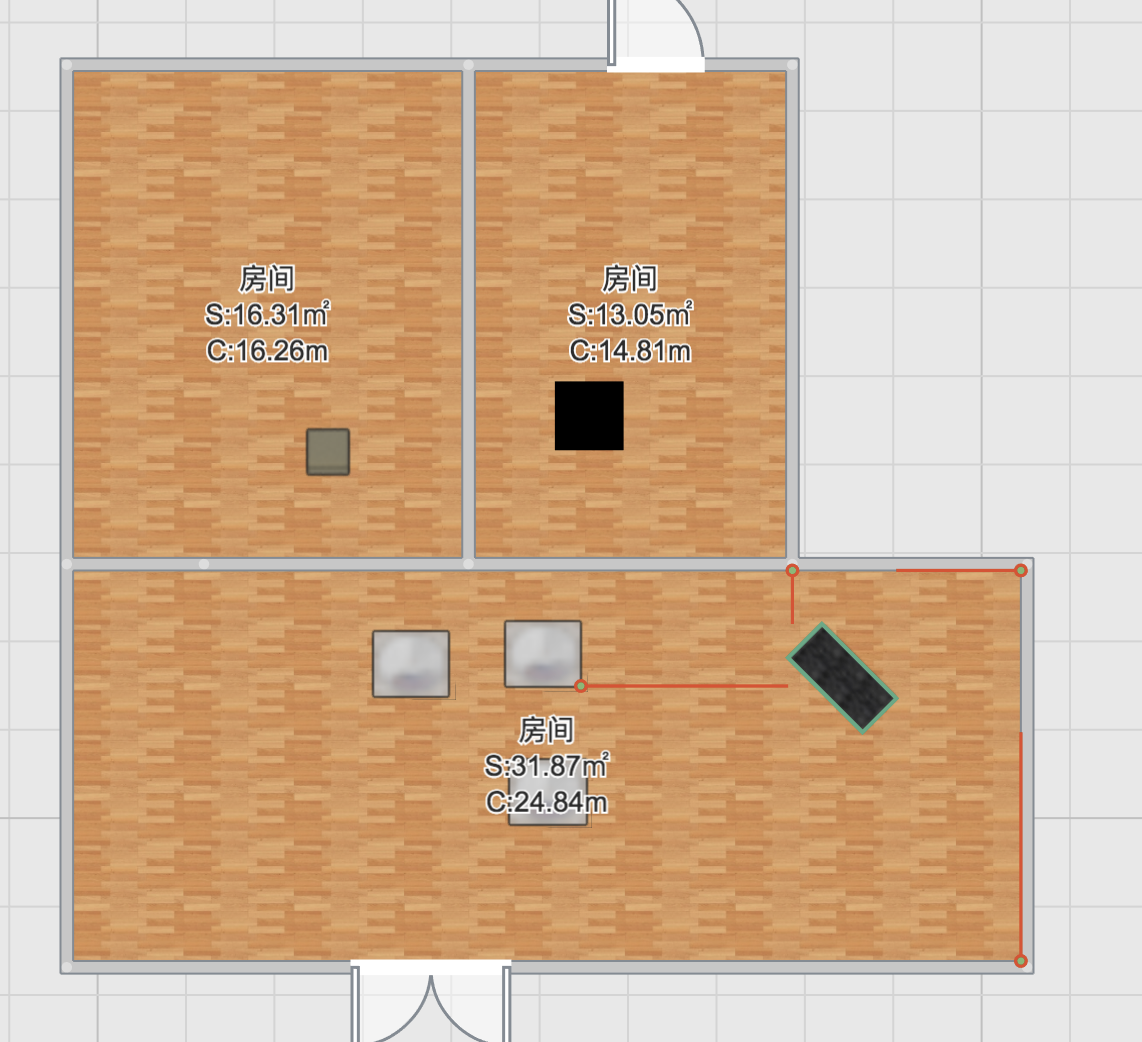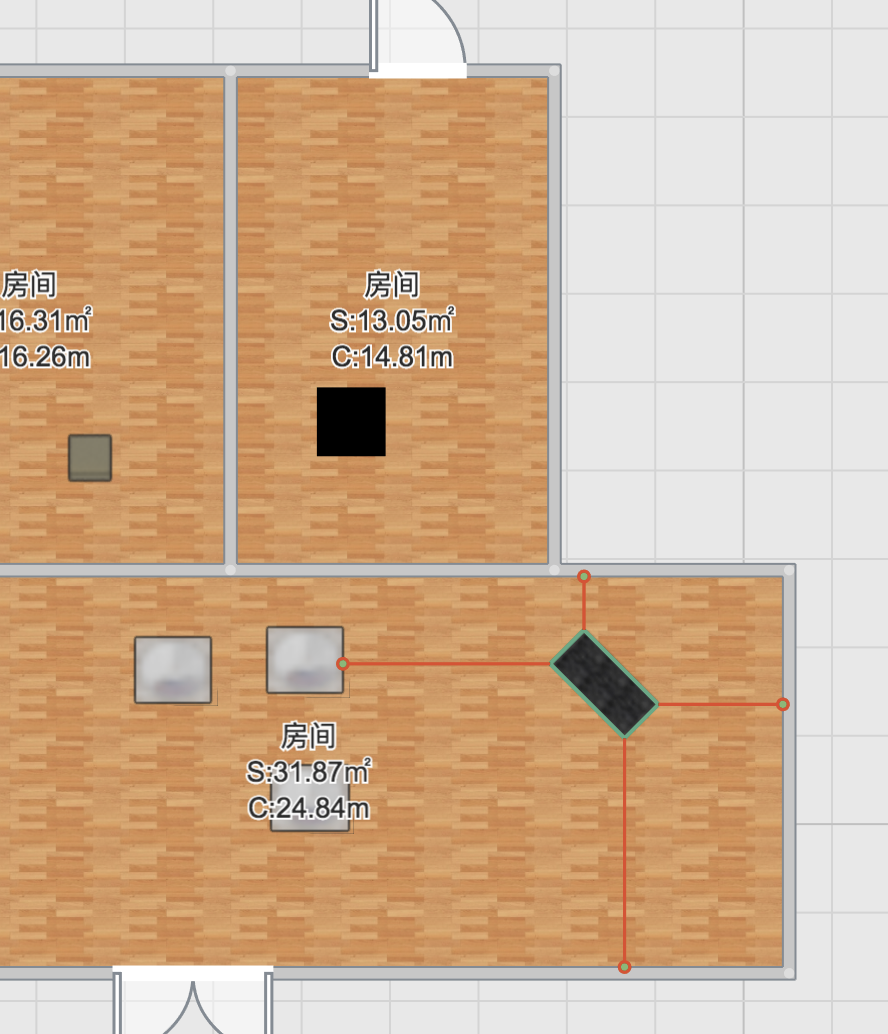### 最终效果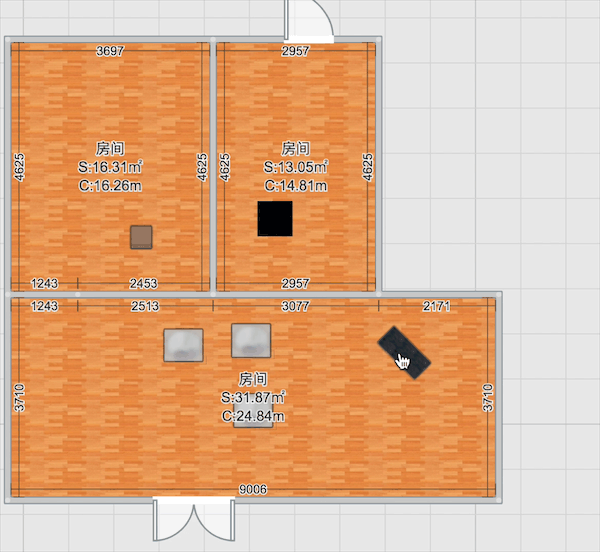### 代码实现

  /**
* 根据模型获取 模型四个方向的最近射线
* @param srcModel
* @return {Line2D[]}
* Created by yee.wang on 2018/9/7
*/
public static getNearLines(srcModel: ModelDataBase): Line2D[] {
const room = ToolRoom.getRoomByPoint(
srcModel.position,
Scene3D.getInstance().homePlan.roomLayer.getDatas() as Room[],
);

if (!room) {
return [];
}

// 当前模型的4个边界点
const edgePoints: Vector2D[] = (() => {
const modelBoundingPoints = ToolModel.getModelPoints(srcModel);

return [
// top:
minBy(modelBoundingPoints, vec => vec.y).clone(),
// right:
maxBy(modelBoundingPoints, vec => vec.x).clone(),
// bottom:
maxBy(modelBoundingPoints, vec => vec.y).clone(),
// left:
minBy(modelBoundingPoints, vec => vec.x).clone(),
];
})();

// 根据当前模型获取到的感应区域
const inductionPolygons = (() => {
const boundingBox = new BoundingBox2D();
edgePoints.forEach(point => boundingBox.expandByPoint(point));

return boundingBox.polygon.getEdges().map(line => {
line = line.translateLeft(1);
line.setLength(line.length - 1); // 缩小范围，防止边界重叠
const rayLine = line.translateLeft(2000);

return new Polygon2D([line.start, line.end, rayLine.end, rayLine.start]);
});
})();

const position2D = new Vector2D(srcModel.position.x, srcModel.position.z);

// 当前房间的除了当前模型的所有模型
const models = room.getModelsInRoom() as ModelDataBase[];
const cubeBoxes = room
.getCubeBoxesInRoom()
.map(cubeBox => ToolCubeBox.makeVirtualModel(cubeBox)) as ModelDataBase[];
const walls = room.walls as Wall[];

const srcPolygon = srcModel.getPolygon();

const nearLines: Line2D[] = [];
inductionPolygons.forEach((polygon, index) => {
const modelLine = new Line2D(polygon.vertices, polygon.vertices);
const rayLine = modelLine.setLength(2000);

const intersectResults = [...models, ...walls, ...cubeBoxes].filter(compare => {
let pos2D: Vector2D = null;
if (compare.position instanceof Vector3D) {
pos2D = new Vector2D(compare.position.x, compare.position.z);
} else {
pos2D = compare.position.clone();
}

// 过滤在线段右侧的物体
if (rayLine.isRight(pos2D)) {
return false;
}

const comparePolygon = compare.getPolygon();
// 过滤与自身相交的模型
if (!!srcPolygon.intersectPolygon(comparePolygon.boundingBox.polygon)) {
return false;
}

return !!polygon.intersectPolygon(comparePolygon);
});

const allPoints = intersectResults.reduce((prev, next) => prev.concat(next.getPolygon().vertices), []);

const nearestPoint = minBy(allPoints, point => position2D.distanceSquared(point));

if (nearestPoint) {
const footPoint = nearestPoint.footPoint(rayLine);

const resultLine = new Line2D(footPoint, nearestPoint);
const originPoint = edgePoints[index];

resultLine.translateBy(originPoint.subtract(resultLine.start) as Vector2D);

nearLines.push(resultLine);
}
});

return nearLines;
}